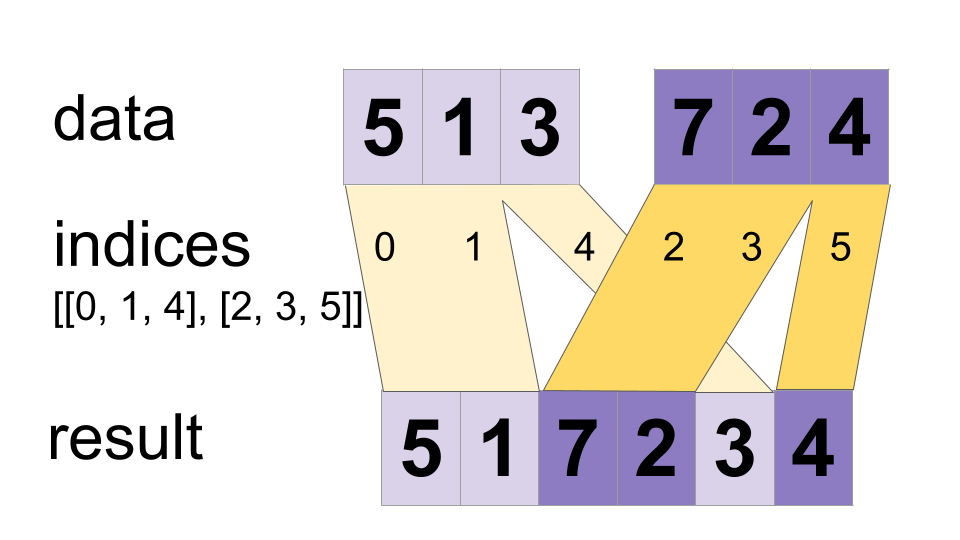Warning: This API is deprecated and will be removed in a future version of TensorFlow after the replacement is stable.

# ParallelDynamicStitch

public final class ParallelDynamicStitch

Interleave the values from the `data` tensors into a single tensor.

Builds a merged tensor such that

``````merged[indices[m][i, ..., j], ...] = data[m][i, ..., j, ...]
``````
For example, if each `indices[m]` is scalar or vector, we have
``````# Scalar indices:
merged[indices[m], ...] = data[m][...]

# Vector indices:
merged[indices[m][i], ...] = data[m][i, ...]
``````
Each `data[i].shape` must start with the corresponding `indices[i].shape`, and the rest of `data[i].shape` must be constant w.r.t. `i`. That is, we must have `data[i].shape = indices[i].shape + constant`. In terms of this `constant`, the output shape is

merged.shape = [max(indices)] + constant

Values may be merged in parallel, so if an index appears in both `indices[m][i]` and `indices[n][j]`, the result may be invalid. This differs from the normal DynamicStitch operator that defines the behavior in that case.

For example:

``````indices = 6
indices = [4, 1]
indices = [[5, 2], [0, 3]]
data = [61, 62]
data = [[41, 42], [11, 12]]
data = [[[51, 52], [21, 22]], [[1, 2], [31, 32]]]
merged = [[1, 2], [11, 12], [21, 22], [31, 32], [41, 42],
[51, 52], [61, 62]]
``````
This method can be used to merge partitions created by `dynamic_partition` as illustrated on the following example:
``````# Apply function (increments x_i) on elements for which a certain condition
# apply (x_i != -1 in this example).
x=tf.constant([0.1, -1., 5.2, 4.3, -1., 7.4])
partitioned_data = tf.dynamic_partition(
partitioned_data = partitioned_data + 1.0
condition_indices = tf.dynamic_partition(
x = tf.dynamic_stitch(condition_indices, partitioned_data)
# Here x=[1.1, -1., 6.2, 5.3, -1, 8.4], the -1. values remain
# unchanged.
``````### Public Methods

 Output asOutput() Returns the symbolic handle of a tensor. static ParallelDynamicStitch create(Scope scope, Iterable> indices, Iterable> data) Factory method to create a class wrapping a new ParallelDynamicStitch operation. Output merged()

## Public Methods

#### public Output<T> asOutput()

Returns the symbolic handle of a tensor.

Inputs to TensorFlow operations are outputs of another TensorFlow operation. This method is used to obtain a symbolic handle that represents the computation of the input.

#### public static ParallelDynamicStitch<T> create(Scope scope, Iterable<Operand<Integer>> indices, Iterable<Operand<T>> data)

Factory method to create a class wrapping a new ParallelDynamicStitch operation.

##### Parameters
scope current scope
##### Returns
• a new instance of ParallelDynamicStitch

#### public Output<T> merged()

[{ "type": "thumb-down", "id": "missingTheInformationINeed", "label":"Missing the information I need" },{ "type": "thumb-down", "id": "tooComplicatedTooManySteps", "label":"Too complicated / too many steps" },{ "type": "thumb-down", "id": "outOfDate", "label":"Out of date" },{ "type": "thumb-down", "id": "samplesCodeIssue", "label":"Samples / code issue" },{ "type": "thumb-down", "id": "otherDown", "label":"Other" }]
[{ "type": "thumb-up", "id": "easyToUnderstand", "label":"Easy to understand" },{ "type": "thumb-up", "id": "solvedMyProblem", "label":"Solved my problem" },{ "type": "thumb-up", "id": "otherUp", "label":"Other" }]